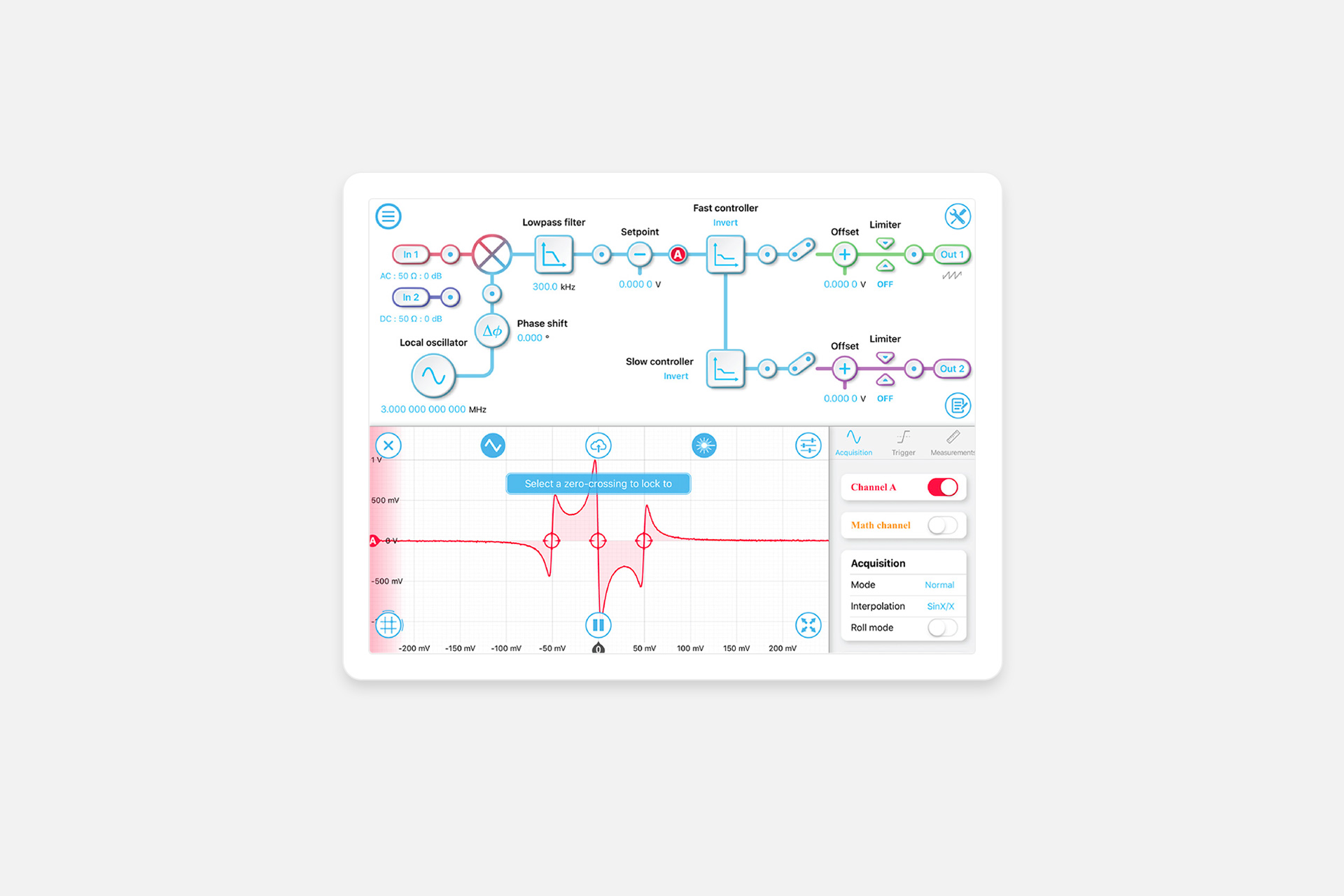Application Notes

# Measuring Allan Deviation — A guide to Allan Deviation with Moku:Lab’s PhasemeterMoku:Lab’s Phasemeter measures phase with up to 6 µradian precision for input signals oscillating between 1 kHz and 200 MHz. Using a digitally implemented phase-locked loop architecture, it provides exceptional dynamic range and precision far exceeding the capabilities of conventional lock-in amplifiers and frequency counters. Moku:Lab’s Phasemeter calculates and plots Allan Deviation, which is a unitless measure of stability, typically used to quantify the stability of clocks and other oscillators. In this guide, we will cover the math and provide an example Allan Deviation calculation with the overlapped variable τ estimator

## Allan Deviation with overlapped variable τ estimators

Allan Deviation is a unitless measure of stability, typically used to quantify the stability of clocks and other oscillators. By definition, Allan Deviation σy is calculated as a function of observation time τ by the following equation:where x is the phase measured over time. In practice, it’s not possible to calculate the expected value over infinite time. Several estimators are used to calculate Allan deviation. Moku:Lab uses the overlapped variable τ estimator to calculate the Allan Deviation, which is defined as:where τ0 is the sampling period of the Phasemeter and N is the number of data points acquired for the input time series. n is an integer multiplier of the sampling period that best estimates the desired τ≅nτ0. xi represents the ith element in the time series.

## Allan Deviation with Moku:Lab’s Phasemeter

In this example, we measured the Allan deviation of a 2 MHz signal with Moku:Lab Phasemeter. The frequency and phase of the signal were measured at 122 Hz for 10 minutes. To display the Allan Deviation, select the “Phase” tab on the top of the Moku display and “Allan Deviation” in the plot area.

Please note, if “Frequency” or “Amplitude” tabs are selected, Moku:Lab will use frequency or amplitude time series as the input for Equation (2).## Python implementation

The function cal_oadev takes the time series, sampling rate and an array of observation time τ as the input. It calculates the overlapped Allan deviation using equation (2) and returns the nτ0 and σy (0 ) as arrays. Required Libraries: NumPy, math

#Import libraries
import numpy as np
import math

tau0 = 1/rate #Calculate the sampling period
dataLength = data.size #Calculate N
dev = np.array([]) #Create empty array to store the output.
actualTau = np.array([])
for i in tauArray:
n = math.floor(i/tau0) #Calculate n given a tau value.
if n == 0:
n = 1 #Use minimal n if tau is less than the sampling period.
currentSum = 0 #Initialize the sum
for j in range(0,dataLength-2*n):
currentSum = (data[j+2*n]-2*data[j+n]+data[j])**2+currentSum
#Cumulate the sum squared
devAtThisTau = currentSum/(2*n**2*tau0**2*(dataLength-2*n))
#Divide by the coefficient
dev = np.append(dev,np.sqrt(devAtThisTau))
actualTau = np.append(actualTau,n*tau0)
return actualTau, dev #Return the actual tau and overlapped Allan deviation

## Example calculation

In this example, we imported the time series we acquired previously on the Moku:Lab and calculated the Allan Deviation with Python scripts. You can find all the raw data and scripts below.

The time series can be found in the “data.xlsx” file. The first column “A” is the measured frequency in Hz, and the second column “B” is the measured phase in cycles. The script “CalculateAllanDeviation.py” reads the excel file and calls the cal_oadev function in the “AllanFunc.py”. The Allan Deviation is plotted as a function of τ in log scales. The Allan Deviation plots matched well between the Moku:Lab and Python scripts. Required Libraries: NumPy, math, pandas, Matplotlib## References

 Land, D. V., A. P. Levick, and J. W. Hand. “The use of the Allan deviation for the measurement of the noise and drift performance of microwave radiometers.” Measurement Science and Technology 18, no. 7 (2007): 1917.

 Allan, David W. “Statistics of atomic frequency standards.” Proceedings of the IEEE 54.2 (1966): 221-230.

 Wikipedia Article: Allan variance, https://wikipedia.org/wiki/Allan_variance, Accessed: 2019-10-30